In this lesson, we'll learn aoubt two properties of financial assets, mean reversion, and co-integration. Based on these properties, we will learn about the trading strategy called, Pairs trading(配对交易). From there, we will examine the more general strategy called, mean reversion trading.

## Mean Reversion

We can plot a stock's price movements against its moving average.We tend to see that when the price increases or decreseses significantly from this moving average, the price then reverts back towards this moving average.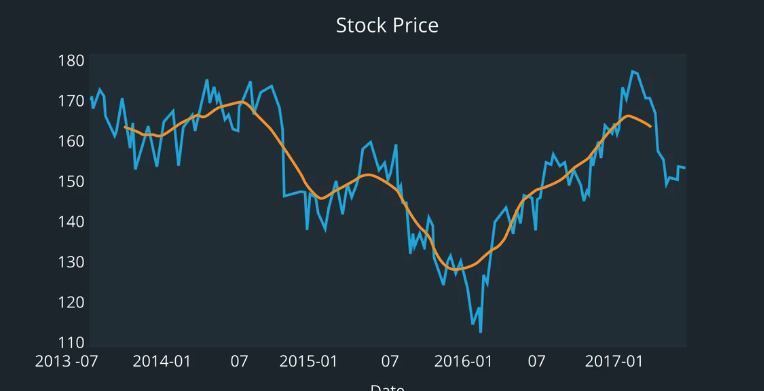To describe a mean reverting process with math, we'll use what's called the Drift and Volatility model.

### Drift and Volatility Model (optional)

he drift and volatility model is also called a Brownian Motion model, and is a type of stochastic volatility model. First, let’s discuss how this relates to the finance industry. Stochastic volatility models are fundamental building blocks for estimating the price of options (calls, puts, swaps) and also bonds. Before creating a model of an option (like a call option, for instance), we first want a model for the movement of its underlying asset (the stock price itself). The movement of the stock price is what the drift and volatility model (brownian motion model) attempts to describe.
The word Brownian Motion refers to the movements of molecules suspended in fluid, since this model was first used in physics and later adapted for finance. So it helps to imagine the stock price as a small particle, drifting through a glass of water, while it’s being bumped around by other particles and molecules. The word “stochastic” is another word for “random”. Stochastic volatility models attempt to represent the movement of a stock price when the volatility of its movements is random. Stochastic volatility models were used to improve upon the work of Black, Scholes and Merton, who came up with the first formula for pricing options.

Now let’s revisit the drift and volatility model and describe what it means.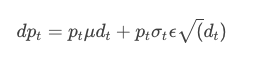First, notice that dpt on the left is referring to the differential of the stock price at time t. This type of equation is called a differential equation, since it describes the change over time of some process, rather than the specific state (stock price) of that series.

The term p_t \mu dtp μdt is the drift term. First, notice that it depends on the value of the stock price at time t (p_t)(p This means that if we compare the movements of two stocks, one that’s priced at $2 per share, and another that’s priced at$1000 per share, the series with the larger price per share is expected to drift (change) more in absolute dollar amounts compared to the other stock. The \muμ term is the expected return of the stock (think average return). Think of the expected return as the expected percent change over a period of time. We usually estimate the expected future return based on historical returns. So if a stock is expected to have a larger percent change per day compared to another, we’d also expect it to drift more (change more) compared to the other stock. This term also includes d_td
, which is the change in time (how much time has passed). If we watched a stock over a period of day versus over one month, we would expect it to drift more over a month, as more time has passed.

### Back to Mean Reversion

Okay, stepping back a bit to relate this to mean reversion. The drift and volatility model is a way to describe phenomena that we observe in real life, such as stock prices. The model assumes that there is a constant drift term with some added randomness, so we can expect that a series will bounce around, but still revert back to its long-term mean.

When two companies have economic links, this implies that their stock prices may also move in a similar manner.
For example, company A sells frozen green peas, and company B cells frozen carrots. Since frozen peas and carrots are often combined and sold together. We can see how an increase in sales of peas and carrots would affect both companies.Most of the time we see the two stocks moving similarly.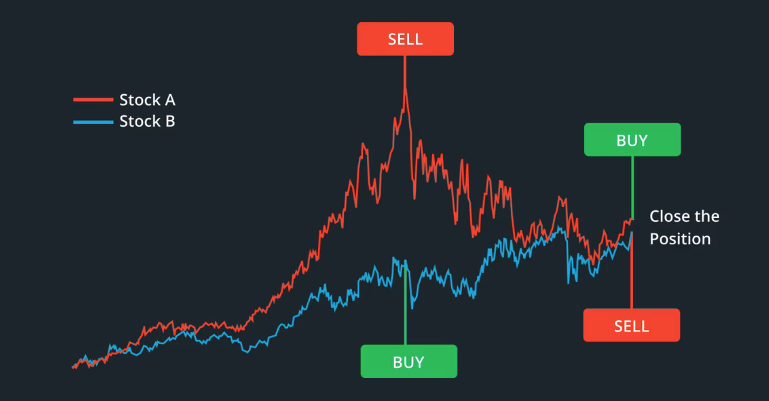Note that with pairs trading, we analyze the original stock price series, and do not convert them to returns or log returns. We’ll get into the details shortly, but let’s just look at an example.

Let’s say $stock_A$ is $2 per share, and $stock_B$ is$3 per share.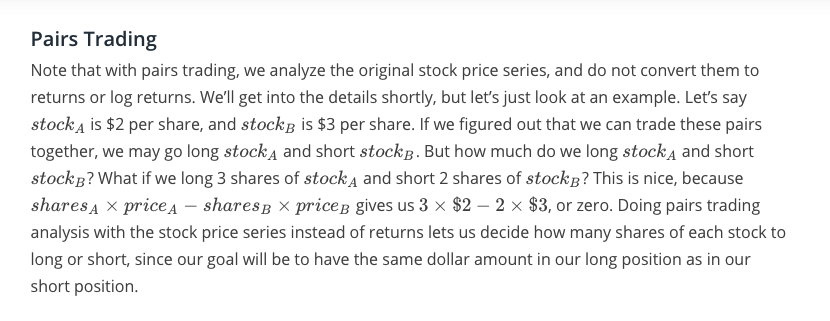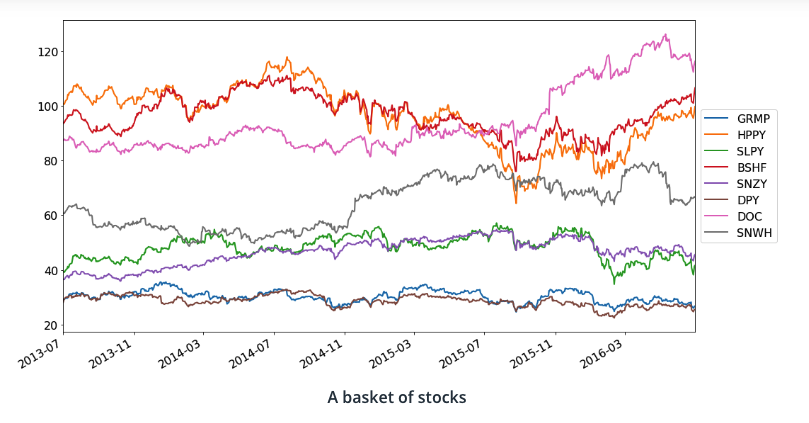Looking at the graph above, mark any pair(s) of stocks that you think will be good candidates for pairs trading.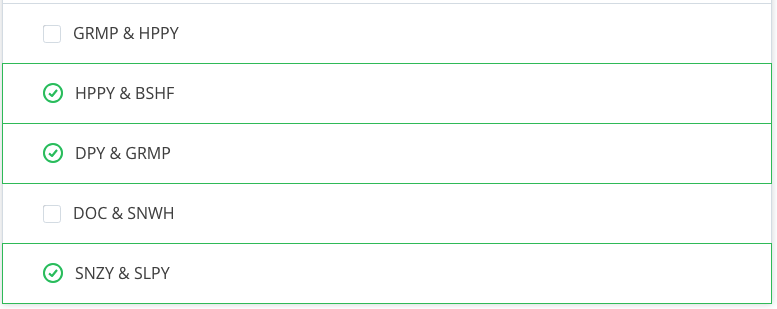## Cointegration(协整)

A way to think about whether two stocks’ time series are cointegrated is to see if some linear combination of their time series forms a stationary series.

let’s say $stock_1$ and $stock_2$ are non-stationary, but w1 x stock1 + w2 x stock2 is a stationary series. Then we can also say that $stock_1$ and $stock_2$ are cointegrated.

### Hedge Ratio(对冲比率)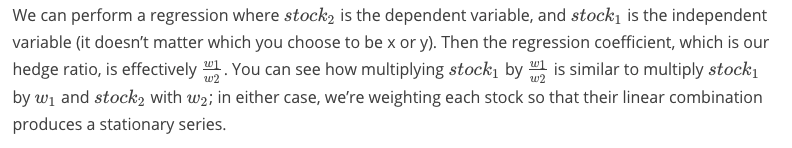### Augmented Dickey Fuller Test

To check if two series are cointegrated, we can use the Augmented Dickey Fuller (ADF) Test. First, let’s get some intuition to see what the ADF test is doing. It’s trying to determine if the linear combination of the two series, (which is also a time series) is stationary.

A series is stationary when its mean and covariance are constant, and also when the autocorrelation between one time period and another only depends on the time duration between them, and not the specific point in time of each observation.